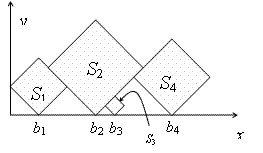• 2017-12-13
• 0
• 1

## Problem:

 Time Limit: 2000MS Memory Limit: 65536K

Description

In this problem, you are given a sequence S1S2, ..., Sn of squares of different sizes. The sides of the squares are integer numbers. We locate the squares on the positive x-y quarter of the plane, such that their sides make 45 degrees with x and y axes, and one of their vertices are on y=0 line. Let bi be the x coordinates of the bottom vertex of Si. First, put S1 such that its left vertex lies on x=0. Then, put S1, (i > 1) at minimum bi such that

i) bi > bi-1 and

ii) the interior of Si does not have intersection with the interior of S1...Si-1.The goal is to find which squares are visible, either entirely or partially, when viewed from above. In the example above, the squares S1S2, and S4 have this property. More formally, Si is visible from above if it contains a point p, such that no square other than Si intersect the vertical half-line drawn from p upwards.

Input

The input consists of multiple test cases. The first line of each test case is n (1 ≤ n ≤ 50), the number of squares. The second line contains n integers between 1 to 30, where the ith number is the length of the sides of Si. The input is terminated by a line containing a zero number.

Output

For each test case, output a single line containing the index of the visible squares in the input sequence, in ascending order, separated by blank characters.

Sample Input

```4
3 5 1 4
3
2 1 2
0
```

Sample Output

```1 2 4
1 3
```

Source

Tehran 2006

## Solution:ps: 注意细节！

## Code: O(n2ST), S为最大边长 [172K, 0MS]

```#include<cstdio>
#include<cstdlib>
#include<cstring>
#include<cmath>
#include<iostream>
#include<algorithm>
using namespace std;

int n, a, b;
int d;  // The max x-coordinate is 3000, which equals to 50 * S

int main(){
while(scanf("%d", &n) && n){
for(register int i = 1; i <= n; i++) scanf("%d", a + i);
// Amplify the length to sqrt(2) times as origin when manipulating below
for(register int i = 1; i <= n; i++){
b[i] = a[i];  // The square cannot get across the y-axis
for(register int j = 1; j < i; j++){
int cur = b[j] + 2 * min(a[i], a[j]);
b[i] = max(b[i], cur);
}
// For each left square, try to put current one at the min position
// The min legal position is the max of former positions
}
bool fir = 1;  // Control output format
for(register int i = 1; i <= n; i++){
for(register int j = b[i] - a[i]; j < b[i] + a[i]; j++) d[j] = 0;
// Initialize the array d[], d[i] controls a region [i, i + 1)
for(register int j = 1; j <= n; j++){
if(a[j] <= a[i]) continue;  // Only larger square can cover smaller square
int inl = max(b[i] - a[i], b[j] - a[j]), inr = min(b[i] + a[i], b[j] + a[j]);
// Covered region might get across [ b[i] - a[i], b[i] + a[i] ), so control it by max/min
if(inl < inr) d[inl]++, d[inr]--;
// Use the method of "difference" to record the coverage
}
int presum = 0;
for(register int j = b[i] - a[i]; j < b[i] + a[i]; j++){
presum += d[j];
if(presum == 0){
if(fir) fir = 0; else printf(" ");
printf("%d", i);
break;  // Once there is a point without coverage, output this square
}
}
}
puts("");
}
return 0;
}
```

#### 评论

•###### pyai 2019-08-30 22:35回复

太棒了，orz

darkleafin.cf
(该域名已过期且被抢注。。)
darkleafin.github.io

49750

https://github.com/Darkleafin

OPEN AT 2017.12.10

Please refresh the page if the code cannot be displayed normally.

https://visualgo.net/en

- Theme by Qzhai